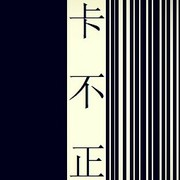[C]该不该 [C]搁下重重[Am]的壳   [Am]       寻[F]找到底 [G]哪里有蓝[C]天   [C] 随[Em]着 轻轻[Em]的风 轻轻[Am]地     [Am]飘     历[F]经的伤 [G]都不感觉[C]疼   [C] {start_of_chorus} 我[C]要 一步[C]一步 往上[Em]爬   [Em]       等待阳[F]光 静静[G]看着它的[C]脸   [C] 小小的[F]天 有[G]大大的梦[Em]想   [Em]       重重的[F]壳 裹着[Dm]轻轻的仰[G]望   [G] {end_of_chorus} {start_of_chorus} 我[C]要 一步[C]一步 往上[Em]爬   [Em]       在最高[F]点 乘着[G]叶片往前[C]飞   [C] 任风吹[F]干 流[G]过的泪和[C]汗   [C]       总有一[F]天 我有[G]属于我的[C]天 {end_of_chorus} 任风吹[F]干 流[G]过的泪和[C]汗   [C]       总有一[F]天 我有[G]属于我的[C]天 总有一[F]天 我有[G]属于我的[C]天 {title:蜗牛} {artist:周杰伦} {author:_wc_wc_}_wc_wc_